Geeks With Blogs

Below is a summary of notes for the exam. Before these will make sense you will have to go through the study material, so this is more just a quick revision summary.

### Format for Declaring a Recursive Statement

You will be asked to compile a recursive definition for some language. In the textbook examples the language was a number based language, but in all the past exams the language has been a text based language so be careful…

You will be asked to state 4 things,

1. Universal Set
2. Generators of Language
3. Appropriate Function on the Universal Set
4. Write a recursive definition using all of the above

For a Text based language the following generally applies…

1. Universal Set (usually a,b or some combination of it) – e.g. {a,b}*
2. Generators of Language (usually the smallest word(s) – be aware of null as a generator) – e.g. aa, bb
3. Appropriate Function – for languages this is typically CONCAT

Assume we were stating a recursive definition for all words containing an a or b over the language contained by {a,b}* – the language call ALLAB

Our recursive definition could look something like this…

Assume a and b are elements of ALLAB,

if w is an element of ALLAB, then

CONCAT(w,a), CONCAT (w,b), CONCAT(a,w) and CONCAT(b,w) are elements of ALLAB

### Format for proving by Induction

Generally how they will ask you to prove something by Induction in the exam will be by using the following format…

• They will ask you a recursive definition for some set
• They will then ask you to formulate the induction principle for the recursive definition
• They will then ask you to apply the induction principle to prove some equation

How to solve these problems…

Assume they ask you to give a recursive definition for all positive integers greater than 2… you would respond…

P is the smallest subset of the set of integers such that

2 is an element of P

if n is an element of P, then n+1 is an element of P

To then formulate the appropriate induction principle it should look something like this…

If a subset A of P is such that 5 is an element of A, and if n is an element of A, then also n+1 is an element of A and A = P

Finally to apply the induction principle to prove some equation you can use the following steps… (e.g. 2n – 3 <= 2^(n-2))

1. State the domain of A (e.g. A = {n is an element of P | 2n – 3 <= 2^(n-2))
2. Show that for the first element fall in the domain of A, the statement hold (e.g. is 5 and element of A)
3. Assume K falls in the domain of A
4. RTP that k+1 falls in the domain of A by manipulating your formula to work back to the assumption of K falling in the domain

An explanation on the principle of induction follows…

With induction you need to remember that if you have a statement that you want to prove true from the natural numbers (1, 2, 3, 4, ...) you can use induction.

Induction involves two real main ideas...

1. The first idea of induction is to prove a base case. This means showing that the statement is true for n=1.
2. Next you show that IF the statement is true for k that implies it is true for k+1.
3. Together these two ideas prove that the statement is true for all natural numbers (1, 2, 3, ...) since if k = 1, then k+2 = 2, if k = 2, k+1=3, etc..

Why does induction work? Well, we know the statement is true for n=1 from the base case. (ONE) now because of (TWO) we know it is true for n=2, and because we know it is true for n=2 because of (TWO) we know it is true for n=3. And because we know it is true for n=3 from (TWO) we know it is true for n=4... and so on….

### Finite Automata

A finite automaton (also known as a finite acceptor) is a collection of three things…

1. A finite set of states, one of which is designated as the initial state, called the start state, and some (maybe none) of which are designated as final states;
2. An alphabet S of possible input letters;
3. A finite set of transitions that tell for each state and for each letter of the input alphabet which state to go to next;

Finite Automata can be represented graphically in many different ways. Below are three different representations of the same FA. Please make note of how the start and final states are represented.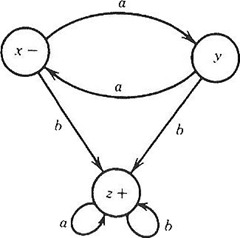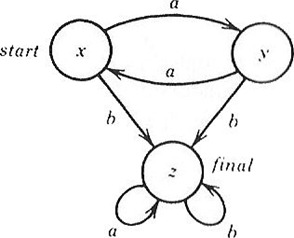Important to note with FA’s

• Every state has as any outgoing edges as there are letters in the alphabet;
• It is possible for a state to have no incoming edges;
• FA’s can only have one start state;
• There are machines where it is not necessary to give the states specific names;
• It is possible if two edges leaves from the same state and go to the same state that they can be put on the same line and separated by a comma (as depicted in the graph below)
• FA are deterministic;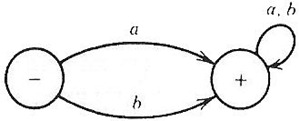### Transition Graphs

A transition graph is a collection of three things…

1. A finite set of states, at least one of which is designated as the start state (-) and some (maybe none) of which are designated as final states (-);
2. An alphabet S of possible input letters from which the input strings are formed;
3. A finite set of transitions (edge labels) that show how to go from some states to some others, based on reading specified substrings of input letters (possibly even the null string);

Important to note with TG’s

• Some states may have no edges coming out of them at all, and some may have thousands of edges;
• TG’s can possibly have more than one start state;
• For a TG to accept anything it must have a final state (else it won’t accept anything including the ^ – Null character)
• Any TG where the start state is also the final state will accept the null character;
• TG are non-deterministic;

Below are two examples of a transition graph…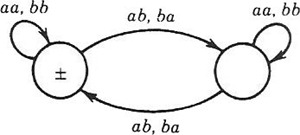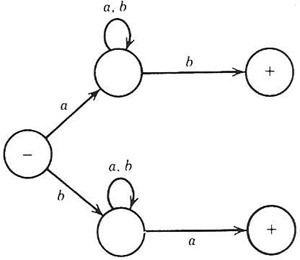### What is Non-Deterministic Machines (Non-Determinism)

Non-deterministic machines are machines where choice becomes a factor in selecting the path through a machine; The machine does not make all its own determinations and the path does not depend on the input alone.

FA’s were deterministic because they required every character in the alphabet to be represented on every edge (so that there was no “choice”). With TG’s, because you can read a series of characters in at once when travelling from one edge to another, depending on how many characters you read and the available edges, it could be valid to move to more than one edge, thus the “choice”.

### Kleene’s Theorem

Basically what Kleene’s theorem proves is that if you have a FA, you can have an equivalent TG and an equivalent Regular Expression and visa versa.

Basic Summary of Theorem

1. Prove that a language defined by a FA can also be defined by a TG
2. Prove that a language defined by a TG can also be defined by a Regular Expression
3. Prove that a language defined by a Regular Expression can also be defined by a FA

Section 1 – Proving a FA can also be defined by a TG

This is pretty elementary as by definition of a FA, it is already a TG

Section 2 – Proving a TG can also be defined by a Regular Expression

This is achieved by simplifying the TG via a series of steps, and then formulating a regular expression from the simplified TG. The following steps should be followed when simplifying a TG.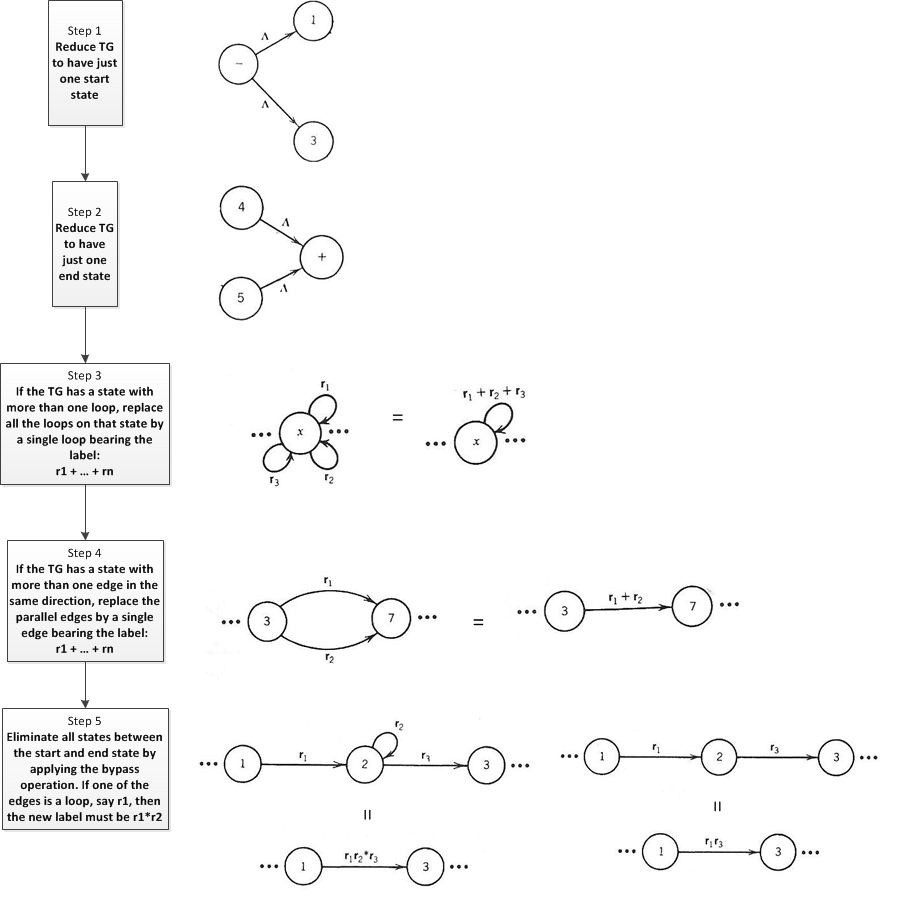Note that the bypass and state elimination operation merely states that if we have three states in a row connected by edges labelled with regular expressions, we can eliminate the middleman and go directly from one outer state to the other by a new edge labelled with a regular expression that is the concatenation of the two previous labels (as illustrated in the diagram above).

There are one or two special cases that one needs to keep in mind when applying the bypass operation. These are illustrated below in the following diagrams….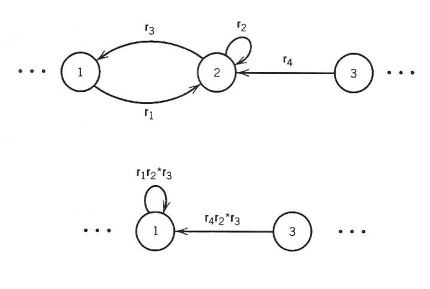Section 3 - Proving a Regular Expression can also be defined by a FA

For the sake of convenience, let us use R to denote the set of regular expressions over S and S to denote the subset of R containing all the regular expressions whose languages can be recognized by FA’s. We want to show R = S.

Rule 1 : The generators of R belong to S (pg. 108-109)

Rule 2 : if r1 is an element of S and r2 is and element of S then r1 + r2 is an element of S (pg. 109-117)

Rule 3 : if r1 is an element of S and r2 is and element of S then r1r2 is an element of S (pg. 117-125)

Rule 4 : if r1 is an element of S then r1* is an element of S (pg. 125-134)

Therefore S = R (i.e. every regular expression defines a language that can be accepted by an FA)

The algorithm for building a FA from a regular expression

Consider a regular expression r that may contain null and any number of other letters…

1. For every letter (and null, if relevant) from r, an FA is built that accepts it;
2. Suppose the factor r1+r2 appears in r, and we have already built two FA’s accepting r1 and r2. Build the FA for r1 + r2;
3. Suppose the factor r1r2 appear in r, and we have already built two FA’s accepting r1 and r2. Build the FA for r1r2;
4. Suppose the factor r1* appears in r, and we have already built an FA accepting r1. Build the FA for r1*;

The actual implementation is explained in the study guide on page 53-54 and should be gone through in detail.

### Nondeterministic FA’s (Special TG)

The basic difference between a FA and a NFA is that in a NFA there does not need be an edge for every letter in S leaving every state and there may be more than one edge with the same label leaving a particular state.

Based on this, every FA can be considered a NFA (but not the other way round).

An NFA is also a special TG – the only sub criteria of a NFA is

• The labels on the edges are single letters (where a TG can have regex)
• It has only one initial state (where a TG can have multiple initial states)

### Regular Languages

Check the textbook for more info… but basically any language that can be defined by a TG, FA or regular expression is a regular language. In the world of languages there are only regular and non-regular languages.

### Transition Tables for FA

FA can be represented by transition tables. Below is a summary of what each element in a transition table represents…

• Each row of the table is the name of one of the states in the FA;
• Each column of the table is a letter of the input alphabet;
• The entries inside the table are the new states that the FA moves into – the transition states;
• Indicated  along the left hand side are the start and final states

For example…

 a b -x y z y x z +z z z

This would equate to the following graphical representation…### Summary Table of Comparison between FA / TG & NFA’s

Below is a summary of some of the properties of each type of machine compared to the others…

 FA TG NFA Number of start states 1 1 or more 1 Number of final states some or none some or none some or none Permissible edge labels letters from S letters from S* letters from S Number of lines leaving each state one edge for each letter in S arbitrary arbitrary Deterministic or not yes no no

### Moore Machines

Below is an example of a Moore machine.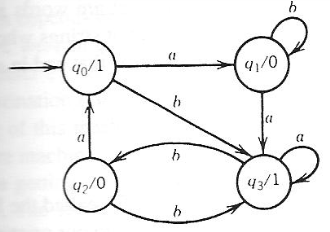• Outputs are done when entering a state;

### Mealy Machines

Below is an example of a Mealy machine.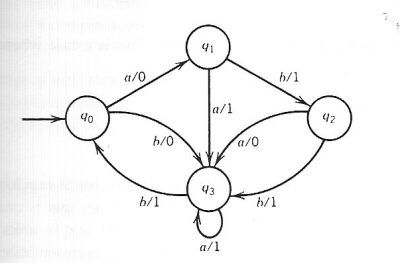• Output is done while travelling along the edges;

### Converting from a Moore to Mealy Machine

Converting from a Moore to Mealy machine is the simpler of the two processes…

• Take any given state in the Moore Machine
• Consider all incoming edges into that state and change them to show the output.
• If the edge was previously labelled a, b & c and the output of the state is 1 on the Moore machine, then on the Mealy machine the edge will be labelled a/1, b/1 ,c/1.
• Apply this for all states in the Moore machine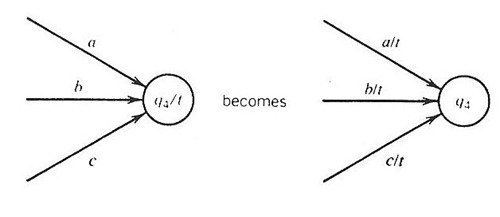Read page 157 / 158 of the textbook for detailed explanation.

### Converting from a Mealy to Moore Machine

Converting from a Mealy machine to a Moore machine is a little more complicated because you can have a situation where two edges might come into the same state but have different output as illustrated in the example below where we have two b incoming edges.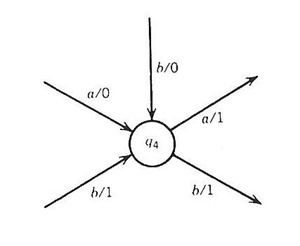To get around this “challenge” we have to make twin copies of the same state (as illustrated below) – one for each output.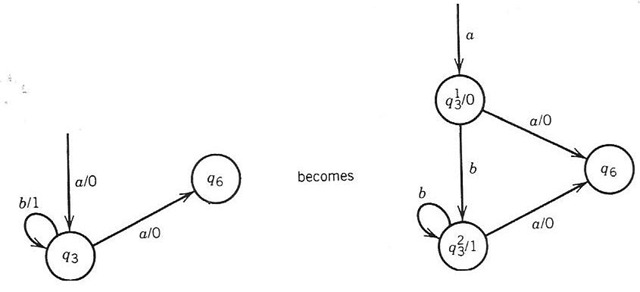If all the incoming edges print the same output we can have one state for the Moore machine.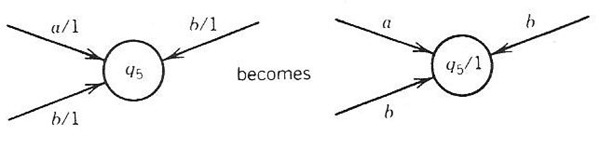One consequence of this algorithm is that an edge that was a loop in a Mealy machine may become two edges in a Moore machine – one edge that is not a loop and one that is (see example below).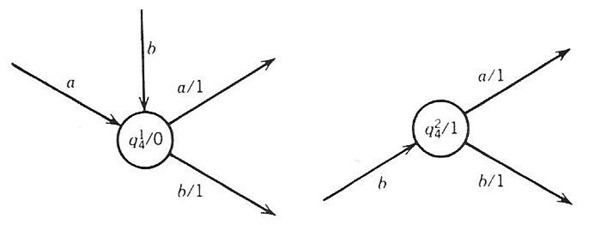The conversion from a Mealy to Moore should be understood properly and I would advise reading page 157 / 158 /159 of the textbook for detailed explanation.

### Difference between Moore & Mealy Machines

The basic difference between these two machines is with the output…

• Output of Moore machine depends only on the current state;
• Output of Mealy machine depends on both the current state and on the input letter being read;

### Pumping Lemma with Length

In the exam all the past papers have asked for the pumping lema with length theorem…

It is stated as follows…

let L be an infinite language accepted by a FA with N states.

Therefore for all w which are elements of L (where length(w) > N)

There are strings X Y & Z with length(Y) > 0

And Length(X) + Length(Y) <= N

Such that w = XYZ

And all strings X(Y^N)Z are elements of L

How do you apply this theorem to prove non regular languages?

• Basically you wont on the proof by contradiction approach, where you assume a language is regular.
• You then state the properties above which will hold for a non regular language
• Once you have done this, you show that by the very definition of the language it will not meet the above properties,
• And thus the language is not regular (Proved by Pumping Lemma)